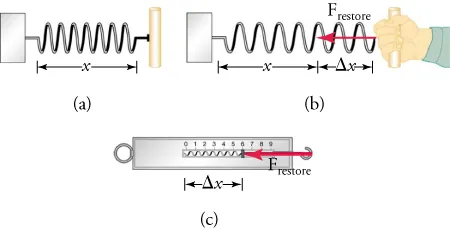Physics

# Extended Response

PhysicsExtended Response

### Extended Response

#### 4.1Force

55.

True or False—When two unequal forces act on a body, the body will not move in the direction of the weaker force.

1. True
2. False
56.

In the figure given, what is Frestore? What is its magnitude?1. Frestore is the force exerted by the hand on the spring, and it pulls to the right.
2. Frestore is the force exerted by the spring on the hand, and it pulls to the left.
3. Frestore is the force exerted by the hand on the spring, and it pulls to the left.
4. Frestore is the force exerted by the spring on the hand, and it pulls to the right.

#### 4.2Newton's First Law of Motion: Inertia

57 .
Two people apply the same force from the same height to throw two identical balls in the air. Will the balls necessarily travel the same distance? Why or why not?
1. No, the balls will not necessarily travel the same distance because the gravitational force acting on them is different.
2. No, the balls will not necessarily travel the same distance because the angle at which they are thrown may differ.
3. Yes, the balls will travel the same distance because the gravitational force acting on them is the same.
4. Yes, the balls will travel the same distance because the angle at which they are thrown may differ.
58.

A person pushes a box from left to right and then lets the box slide freely across the floor. The box slows down as it slides across the floor. When the box is sliding freely, what is the direction of the net external force?

1. The net external force acts from left to right.
2. The net external force acts from right to left.
3. The net external force acts upward.
4. The net external force acts downward.

#### 4.3Newton's Second Law of Motion

59.

A 55-kg lady stands on a bathroom scale inside an elevator. The scale reads 70 kg. What do you know about the motion of the elevator?

1. The elevator must be accelerating upward.
2. The elevator must be accelerating downward.
3. The elevator must be moving upward with a constant velocity.
4. The elevator must be moving downward with a constant velocity.
60.

True or False—A skydiver initially accelerates in his jump. Later, he achieves a state of constant velocity called terminal velocity. Does this mean the skydiver becomes weightless?

1. Yes
2. No

#### 4.4Newton's Third Law of Motion

61.

How do rockets propel themselves in space?

1. Rockets expel gas in the forward direction at high velocity, and the gas, which provides an internal force, pushes the rockets forward.
2. Rockets expel gas in the forward direction at high velocity, and the gas, which provides an external force, pushes the rockets forward.
3. Rockets expel gas in the backward direction at high velocity, and the gas, which is an internal force, pushes the rockets forward.
4. Rockets expel gas in the backward direction at high velocity, and the gas, which provides an external force, pushes the rockets forward.
62.

Are rockets more efficient in Earth’s atmosphere or in outer space? Why?

1. Rockets are more efficient in Earth’s atmosphere than in outer space because the air in Earth’s atmosphere helps to provide thrust for the rocket, and Earth has more air friction than outer space.
2. Rockets are more efficient in Earth’s atmosphere than in outer space because the air in Earth’s atmosphere helps to provide thrust to the rocket, and Earth has less air friction than the outer space.
3. Rockets are more efficient in outer space than in Earth’s atmosphere because the air in Earth’s atmosphere does not provide thrust but does create more air friction than in outer space.
4. Rockets are more efficient in outer space than in Earth’s atmosphere because the air in Earth’s atmosphere does not provide thrust but does create less air friction than in outer space.
Order a print copy

As an Amazon Associate we earn from qualifying purchases.